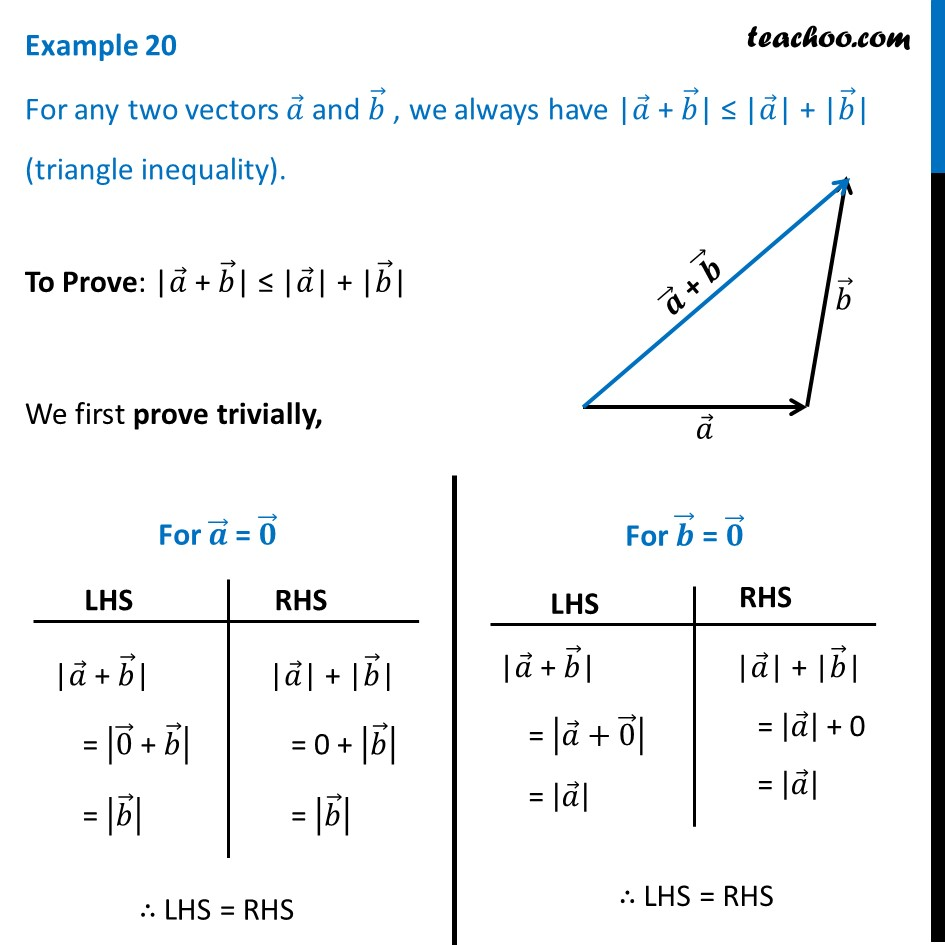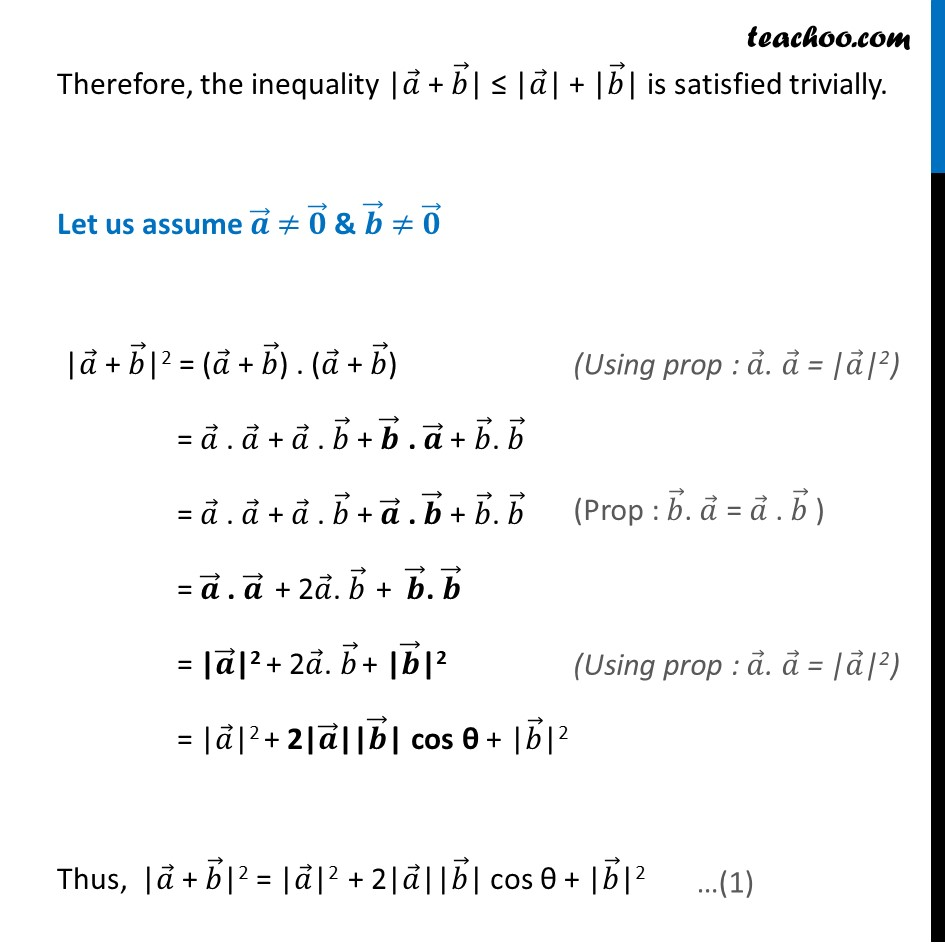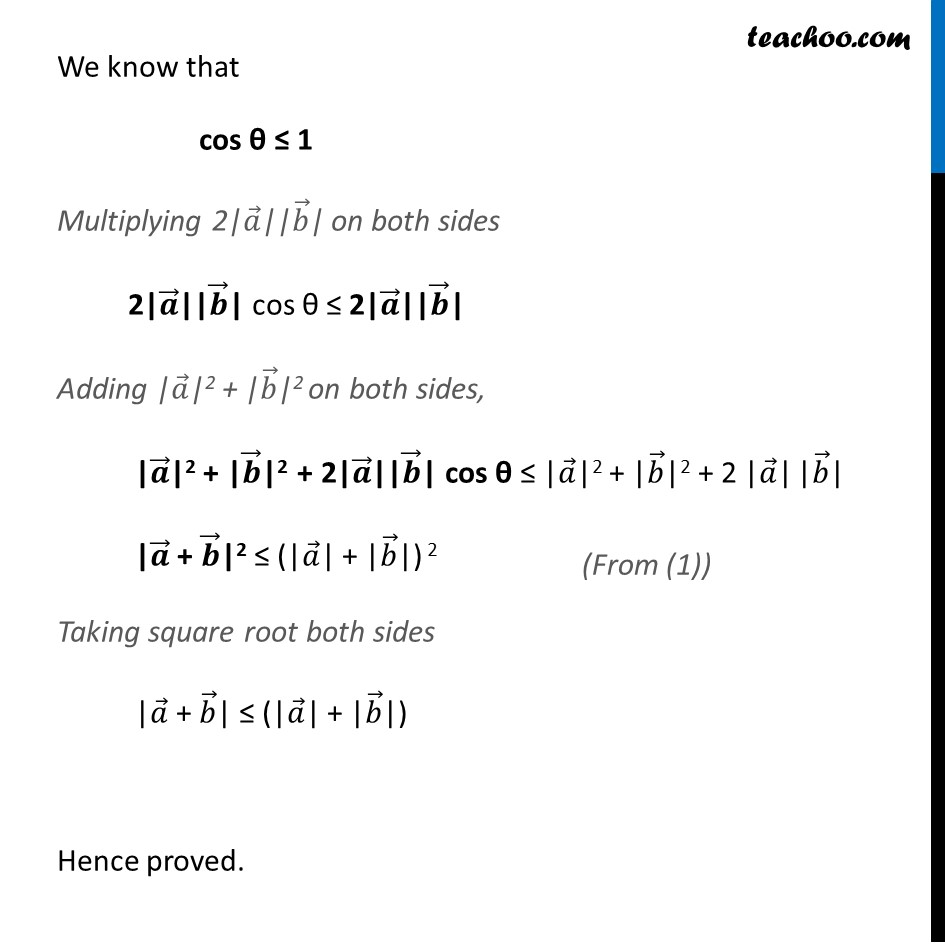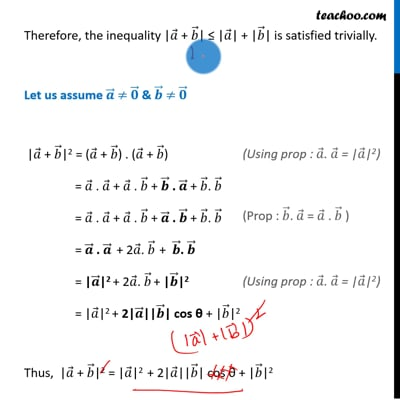Examples

Chapter 10 Class 12 Vector Algebra
Serial order wiseThis video is only available for Teachoo black users

Learn in your speed, with individual attention - Teachoo Maths 1-on-1 Class

### Transcript

Example 20 For any two vectors 𝑎 ⃗ and 𝑏 ⃗ , we always have |𝑎 ⃗ + 𝑏 ⃗| ≤ |𝑎 ⃗| + |𝑏 ⃗| (triangle inequality).To Prove: |𝑎 ⃗ + 𝑏 ⃗| ≤ |𝑎 ⃗| + |𝑏 ⃗| We first prove trivially, |𝑎 ⃗ + 𝑏 ⃗| = |0 ⃗" + " 𝑏 ⃗ | = |𝑏 ⃗ | "|" 𝑎 ⃗"| + |" 𝑏 ⃗"|" = 0 + |𝑏 ⃗ | = |𝑏 ⃗ | |𝑎 ⃗ + 𝑏 ⃗| = |𝑎 ⃗+0 ⃗ | = |𝑎 ⃗ | "|" 𝑎 ⃗"| + |" 𝑏 ⃗"|" = |𝑎 ⃗ | + 0 = |𝑎 ⃗ | Therefore, the inequality |𝑎 ⃗ + 𝑏 ⃗| ≤ |𝑎 ⃗| + |𝑏 ⃗| is satisfied trivially. Let us assume 𝒂 ⃗ ≠ 𝟎 ⃗ & 𝒃 ⃗ ≠ 𝟎 ⃗ |𝑎 ⃗ + 𝑏 ⃗|2 = (𝑎 ⃗ + 𝑏 ⃗) . (𝑎 ⃗ + 𝑏 ⃗) = 𝑎 ⃗ . 𝑎 ⃗ + 𝑎 ⃗ . 𝑏 ⃗ + 𝒃 ⃗ . 𝒂 ⃗ + 𝑏 ⃗. 𝑏 ⃗ = 𝑎 ⃗ . 𝑎 ⃗ + 𝑎 ⃗ . 𝑏 ⃗ + 𝒂 ⃗ . 𝒃 ⃗ + 𝑏 ⃗. 𝑏 ⃗ = 𝒂 ⃗ . 𝒂 ⃗ + 2𝑎 ⃗. 𝑏 ⃗ + 𝒃 ⃗. 𝒃 ⃗ = |𝒂 ⃗|2 + 2𝑎 ⃗. 𝑏 ⃗ + |𝒃 ⃗|2 = |𝑎 ⃗|2 + 2|𝒂 ⃗||𝒃 ⃗| cos θ + |𝑏 ⃗|2 Thus, |𝑎 ⃗ + 𝑏 ⃗|2 = |𝑎 ⃗|2 + 2|𝑎 ⃗||𝑏 ⃗| cos θ + |𝑏 ⃗|2 (Using prop : 𝑎 ⃗. 𝑎 ⃗ = |𝑎 ⃗|2) (Using prop : 𝑎 ⃗. 𝑎 ⃗ = |𝑎 ⃗|2) We know that cos θ ≤ 1 Multiplying 2|𝑎 ⃗||𝑏 ⃗| on both sides 2|𝒂 ⃗||𝒃 ⃗| cos θ ≤ 2|𝒂 ⃗||𝒃 ⃗| Adding |𝑎 ⃗|2 + |𝑏 ⃗|2 on both sides, |𝒂 ⃗|2 + |𝒃 ⃗|2 + 2|𝒂 ⃗||𝒃 ⃗| cos θ ≤ |𝑎 ⃗|2 + |𝑏 ⃗|2 + 2 |𝑎 ⃗| |𝑏 ⃗| |𝒂 ⃗ + 𝒃 ⃗|2 ≤ (|𝑎 ⃗| + |𝑏 ⃗|) 2 Taking square root both sides |𝑎 ⃗ + 𝑏 ⃗| ≤ (|𝑎 ⃗| + |𝑏 ⃗|) Hence proved.